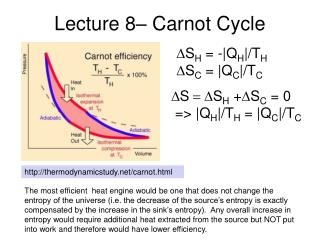# Lecture 8– Carnot Cycle - PowerPoint PPT PresentationDownload PresentationLecture 8– Carnot Cycle

Lecture 8– Carnot Cycle
Download Presentation## Lecture 8– Carnot Cycle

- - - - - - - - - - - - - - - - - - - - - - - - - - - E N D - - - - - - - - - - - - - - - - - - - - - - - - - - -
##### Presentation Transcript

1. Lecture 8– Carnot Cycle DSH = -|QH|/TH DSC = |QC|/TC DS = DSH +DSC = 0 => |QH|/TH = |QC|/TC http://thermodynamicstudy.net/carnot.html The most efficient heat engine would be one that does not change the entropy of the universe (i.e. the decrease of the source’s entropy is exactly compensated by the increase in the sink’s entropy). Any overall increase in entropy would require additional heat extracted from the source but NOT put into work and therefore would have lower efficiency.

2. An important engine to know about is the Stirling engine, which achieves the Carnot efficiency (at least in an idealized form, this is, not surprisingly, never achieved in practice). The diagrams below are from Zemansky’s book “Heat and Thermodynamics”. The wikipedia page on this has some nice applets that show how practical Stirling engines can be built. http://en.wikipedia.org/wiki/Stirling_engine In this style of engine, R is called the Regenerator, it accepts heat from the fluid and then gives it back. There are other variants of the same basic idea (a cycle with two “isochoric” processes linked by two “isothermal” processes).

3. Chapter 3 problems (examples) 8. Refrigerator. When run backward, a Carnot cycle provides an ideal refrigerator: the cycle extracts energy from the cold reservoir and dumps it into the hot reservoir. The left-hand side of figure 3.4 illustrates this cyclic process. Work from an external source is required to run the refrigerator, and the energy associated with that work is also dumped into the hot reservoir. A room air conditioner is fundamentally a refrigerator and the question below examines it:

4. Lecture 8– Otto Cycle (simplified) Isochoric (constant volume) heating and cooling, connected by adiabatic expansions and compressions. The Internal combustion engine (ICE) is approximated by the Otto Cycle (not really closely, since in the ICE, the working fluid is not contained; also the exhaust and intake strokes are combined into the step from 2 to 3 in the idealized cycle)

5. Chapter 3 problems (examples) The CRC handbook of Chemistry and Physics (81st edition) gives the heat capacity of diamond (due to the vibrational motion of the atoms) as: CV(T) = (12/5)p4 N kB (T/q)3 Where q is a constant, and for diamond is equal to 2340 K (this is the so-called Debye temperature for the solid and we will see more about this later). Determine the entropy of 1 mole of diamond at room temperature (300K). How does the above heat capacity compare to the “equi-partition theorem” result which suggests that the heat capacity should be 1/2kB for each “quadratic” degree of freedom in the expression for the energy of the system?

6. Chapter 3 problems (examples) The CRC handbook of Chemistry and Physics (81st edition) gives the heat capacity of diamond (due to the vibrational motion of the atoms) as: CV(T) = (12/5)p4 N kB (T/q)3 Where q is a constant, and for diamond is equal to 2340 K (this is the so-called Debye temperature for the solid and we will see more about this later). Determine the entropy of 1 mole of diamond at room temperature (300K). How does the above heat capacity compare to the “equi-partition theorem” result which suggests that the heat capacity should be 1/2kB for each “quadratic” degree of freedom in the expression for the energy of the system? For the second part of the question, we note that at 300 K, the above expression gives: 0.49 NkB (or 4.1 J/K.mole) for the heat capacity, and the classical result gives 3 NkB.(0.5kB for each quadratic degree of freedom, or 24.9 J/K.mole).

7. Chapter 3 problems (examples)

8. Definition of the Kelvin Scale • The TRIPLE POINT OF WATER (the only point in Density-Pressure,-Temperature space where solid, liquid, and gaseous H2O can coexist in equilibrium) is defined to be at 273.16 K. Since 0K is defined to be absolute zero, this defines the Kelvin scale completely. • The normal melting point of water (0oC) occurs at a higher pressure (101.3 kPa rather than 612 Pa) and slightly lower temperature (273.15 K). • We’ll talk more about this diagram in the last few weeks of the semester. 273.15K 273.16K http://boomeria.org/chemlectures/textass2/fig17-9.jpg

9. States viewed in k-space Green box: (0,0,1) Blue box: (0,0,0) Orange box: (0,1,0) nz 2 1 ny 0 k = nxex + nyey + nzez nx

10. Single-particle states in a box The number of states with energy less than a certain value, is equal to the “volume of a sphere” of appropriate radius, divided by the “volume” per state. On the left we show the case for two dimensions (see HW for this week).